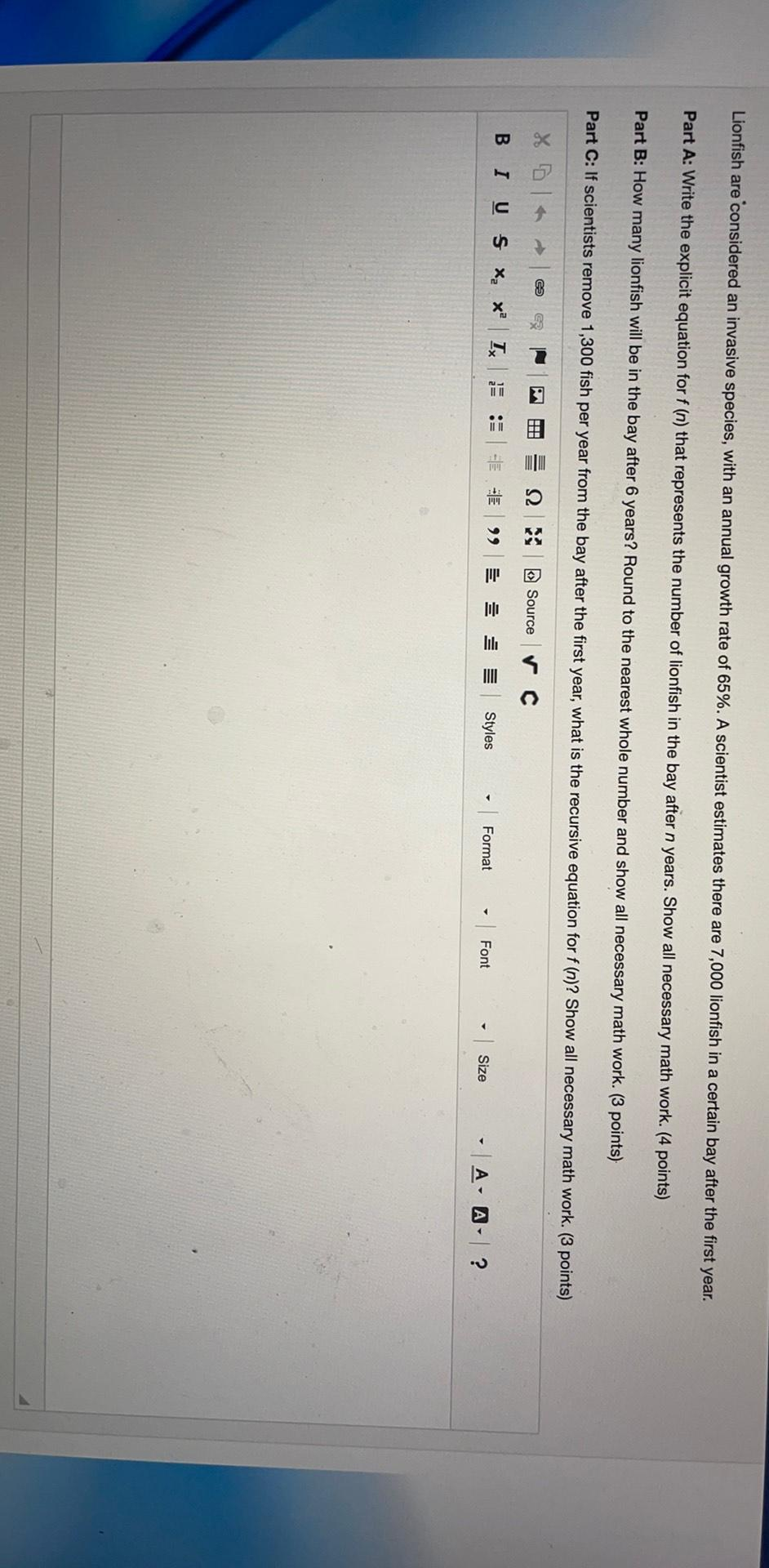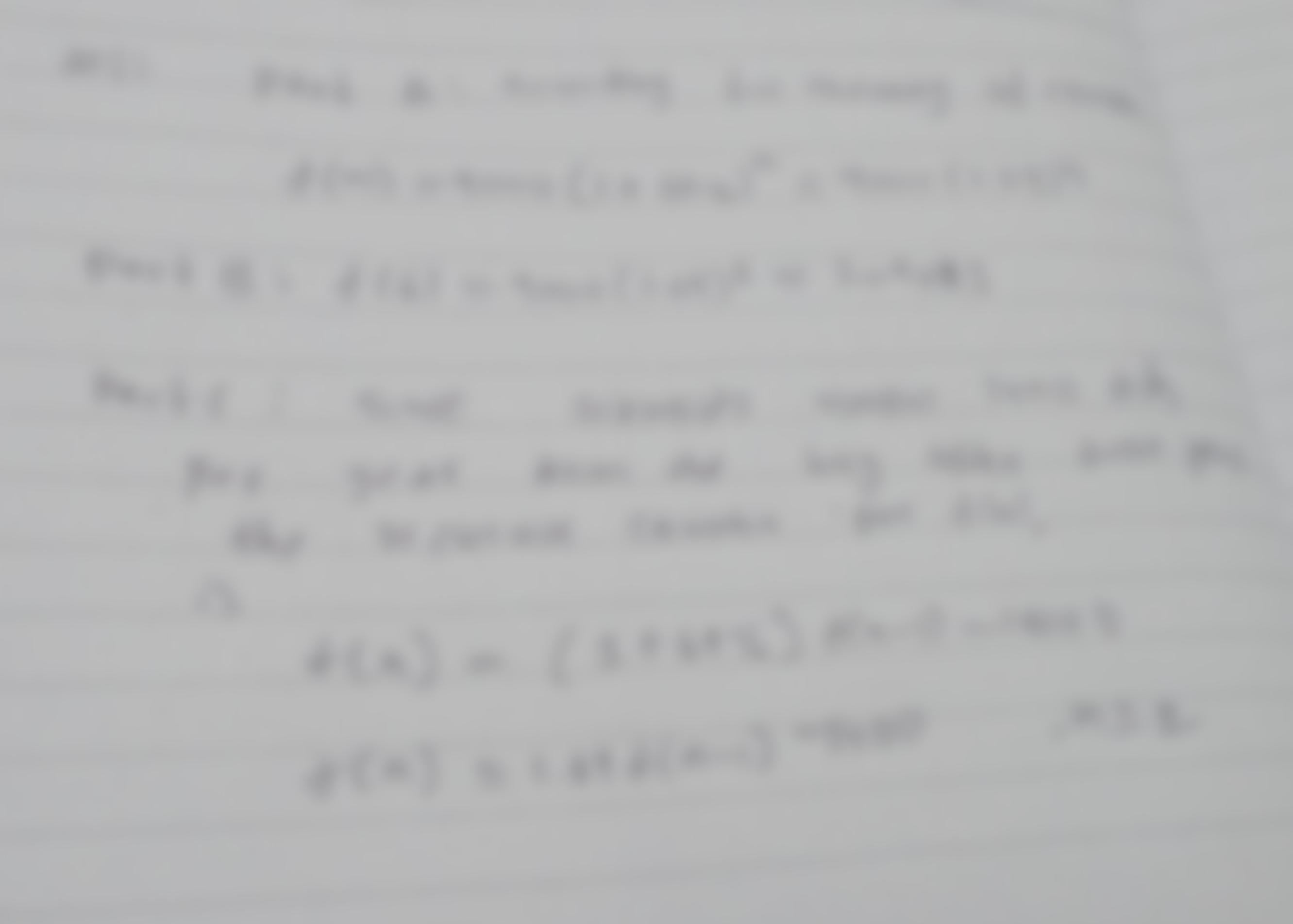Question:

Lionfish are considered an invasive species, with an annual growth rate of 65%. A scientist estimates there are 7,000 lionfish iLionfish are considered an invasive species, with an annual growth rate of 65%. A scientist estimates there are 7,000 lionfish in a certain bay after the first year. Part A: Write the explicit equation for f (n) that represents the number of lionfish in the bay after n years. Show all necessary math work. (4 points) Part B: How many lionfish will be in the bay after 6 years? Round to the nearest whole number and show all necessary math work. (3 points) Part C: If scientists remove 1,300 fish per year from the bay after the first year, what is the recursive equation for f(n)? Show all necessary math work. (3 points) X 1 ce E 12 Source ✓c B 1 0 5 X, xº Ix • Ele 99 Styles Format | Font Size A- A